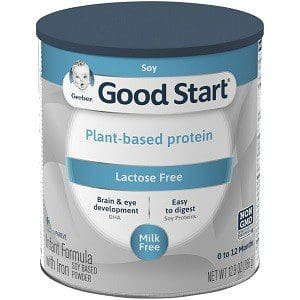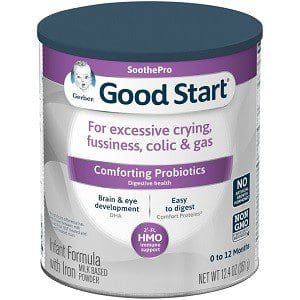### Effective October 1, 2019, there will be a change to the standard infant formula provided by the Vermont WIC Nutrition Program.

• Gerber Good Start Gentle and Gerber Good Start Soy will be the primary WIC milk-based and soy-based formulas replacing Similac Advance and Isomil Soy.
• Gerber Good Start Soothe will be available without a prescription replacing Similac Fuss & Gas, Similac Total Comfort and Similac for Spit up

### Effective October 2019:No Longer WIC Eligible in Vermont: Similac Advance

New Formula: Gerber Good Start Gentle

• Milk BasedNo Longer WIC Eligible in Vermont: Similac Isomil Soy

New Formula: Gerber Good Start Soy

• Soy-Based

• Kosher and HalaiNo Longer WIC Eligible in Vermont: Similac Sensitive Fuss & Guss, Smiliac Total Comfort, Similac for Spit Up

New Formula: Gerber Good Start Soothe

• Milk-based with smaller proteins for easier digestion. Helps with colic, fussiness & gas, and spit up

## Changing Your Baby to a New Formula

Most babies will switch to a new formula without any problems. Some parents may notice minor changes in feeding or pooping patterns when switching their babies to a new formula.

Slowly mix the old formula with the new formula over a period of about a week.

• Prepare some of the new formula and some of the old formula separately, then mix the two prepared formulas together in the amounts in the chart below.
• Look for the amount of formula your baby usually drinks at a feeding in the left-hand column.
• Using columns to the right, mix the amount of current formula with the amount of the new formula.
• Use less of the old formula and more of the new formula each day over the course of the week.
##### If Your Baby Drinks a 4 oz Bottle of Formula

Days 1 and 2

3 oz current formula + 1 oz new formula

Days 3 and 4

2 oz current formula + 2 oz new formula

Days 5 and 6

1 oz current Formula + 3 oz new formula

Day 7

4 oz new formula

##### If Your Baby Drinks a 6 oz Bottle of Formula

Days 1 and 2

4 oz current formula + 2 oz new formula

Days 3 and 4

3 oz current formula = 3 oz new formula

Days 5 and 6

2 oz current Formula + 4 oz new formula

Day 7

6 oz new formula

##### If Your Baby Drinks a 8 oz Bottle of Formula

Days 1 and 2

5 oz current formula + 1 oz new formula

Days 3 and 4

4 oz current formula + 34 oz new formula

Days 5 and 6

2 oz current Formula + 6 oz new formula

Day 7

8 oz new formulaEnglish Español Tiếng Việt العربية Afsoomaali 简体中文